Shared Concepts and Topics

Syntax: LSMESTIMATE Statement

• LSMESTIMATE model-effect <'label'> values <divisor=n> <, <'label'> values <divisor=n>> <, …></ options>;

In contrast to a multirow estimate in the ESTIMATE statement, you specify only a single effect in the LSMESTIMATE statement. The row labels are optional and follow the model-effect specification. For example, the following statements fit a split-split-plot design and compare the average of the third and fourth LS-mean of the whole-plot factor A to the first LS-mean of the factor:

proc glimmix;
class a b block;
model y = a b a*b /  s;
random int a / sub=block;
lsmestimate A 'a1 vs avg(a3,a4)' 2 0 -1 -1 divisor=2;
run;

The order in which coefficients are assigned to the least squares means corresponds to the order in which they are displayed in the "Least Squares Means" table. You can use the ELSM option to see how coefficients are matched to levels of the fixed effect.

The optional divisor=n specification enables you to assign a separate divisor to each row of the LSMESTIMATE. You can also assign divisor values through the DIVISOR= option. See the description of the DIVISOR= option that follows for the interaction between the two ways of specifying divisors.

Table 19.25 summarizes important options in the LSMESTIMATE statement. All LSMESTIMATE options are subsequently discussed in alphabetical order.

Table 19.25: LSMESTIMATE Statement Options

Option

Description

Construction and Computation of LS-Means

Modifies covariate values in computing LS-means

Computes separate margins

Specifies a list of values to divide the coefficients

Specifies the weighting scheme for LS-means computation as determined by a data set

Tunes estimability checking

Degrees of Freedom and p-values

Determines the method for multiple-comparison adjustment of LS-means differences

ALPHA=Determines the confidence level ()

Performs one-sided, lower-tailed inference

Adjusts multiple-comparison p-values further in a step-down fashion

Specifies values under the null hypothesis for tests

Performs one-sided, upper-tailed inference

Statistical Output

Constructs confidence limits for means and mean differences

Displays the correlation matrix of LS-means

Displays the covariance matrix of LS-means

Prints thematrix

Prints thematrix

Produces a joint F or chi-square test for the LS-means and LS-means differences

Requests graphs of means and mean comparisons

Specifies the seed for computations that depend on random numbers

Generalized Linear Modeling

Specifies how to construct estimable functions with multinomial data

Exponentiates and displays LS-means estimates

Computes and displays estimates and standard errors of LS-means (but not differences) on the inverse linked scale

You can specify the following options in the LSMESTIMATE statement after a slash (/):

specifies how denominator degrees of freedom are determined when p-values and confidence limits are adjusted for multiple comparisons with the ADJUST= option. When you do not specify the ADJDFE= option or when you specify ADJDFE=SOURCE, the denominator degrees of freedom for multiplicity-adjusted results are the denominator degrees of freedom for the LS-mean effect in the "Type III Tests of Fixed Effects" table.

The ADJDFE=ROW setting is useful if you want multiplicity adjustments to take into account that denominator degrees of freedom are not constant across estimates. For example, this can be the case when the denominator degrees of freedom are computed by the Satterthwaite or Kenward-Roger method (Kenward and Roger, 1997) in a mixed model.

The ADJDFE= option is not supported by the procedures that perform chi-square-based inference (GENMOD, LOGISTIC, PHREG and SURVEYLOGISTIC).

requests a multiple comparison adjustment for the p-values and confidence limits for the LS-mean estimates. The adjusted quantities are produced in addition to the unadjusted p-values and confidence limits. Adjusted confidence limits are produced if the CL or ALPHA= option is in effect. For a description of the adjustments, see Chapter 45: The GLM Procedure, and Chapter 67: The MULTTEST Procedure, in addition to the documentation for the ADJUST= option in the LSMEANS statement.

Not all adjustment methods of the LSMEANS statement are available for the LSMESTIMATE statement. Multiplicity adjustments in the LSMEANS statement are designed specifically for differences of least squares means.

If you specify the STEPDOWN option, the p-values are further adjusted in a step-down fashion.

ALPHA=number

requests that a t type confidence interval be constructed for each of the LS-means with confidence level 1 – number. The value of number must be between 0 and 1; the default is 0.05.

AT variable=value
AT (variable-list)=(value-list)
AT MEANS

modifies the values of the covariates used in computing LS-means. See the AT option in the LSMEANS statement for details.

BYLEVEL

requests that the procedure compute separate margins for each level of the LSMEANS effect.

The standard LS-means have equal coefficients across classification effects. The BYLEVEL option changes these coefficients to be proportional to the observed margins. This adjustment is reasonable when you want your inferences to apply to a population that is not necessarily balanced but has the margins observed in the input data set. In this case, the resulting LS-means are actually equal to raw means for fixed-effects models and certain balanced random-effects models, but their estimated standard errors account for the covariance structure that you have specified. If a WEIGHT statement is specified, the procedure uses weighted margins to construct the LS-means coefficients.

If the AT option is specified, the BYLEVEL option disables it.

CATEGORY=category-options

specifies how to construct estimates and multiplicity corrections for models with multinomial data (ordinal or nominal). This option is also important for constructing sets of estimable functions for F tests with the JOINT option.

The category-options indicate how response variable levels are treated in constructing the estimable functions. Possible value for the category-options are the following:

JOINT

computes the estimable functions for every nonredundant category and treats them as a set. For example, a three-row LSMESTIMATE statement in a model with three response categories leads to six estimable functions.

SEPARATE

computes the estimable functions for every nonredundant category in turn. For example, a three-row LSMESTIMATE statement in a model with three response categories leads to two sets of three estimable functions.

quoted-value-list

computes the estimable functions only for the specified list of values. The list must consist of formatted values of the response categories.

For further details about using the CATEGORY= option in models for multinomial data, see the documentation for the CATEGORY= option in the ESTIMATE statement.

The CATEGORY= option is supported only by the procedures that support generalized linear modeling (GENMOD, LOGISTIC, and SURVEYLOGISTIC) and by PROC PLM when it is used to perform statistical analyses on item stores that were created by these procedures.

CHISQ

requests that chi-square tests be performed in addition to F tests, when you request an F test with the JOINT option. This option has no effect in procedures that produce chi-square statistics by default.

CL

requests that t type confidence limits be constructed for each of the LS-means. The confidence level is 0.95 by default; this can be changed with the ALPHA= option. If you specify an ADJUST= option, then the confidence limits are adjusted for multiplicity. But if you also specify STEPDOWN , then only p-values are step-down adjusted, not the confidence limits.

CORR

displays the estimated correlation matrix of the linear combination of the least squares means.

COV

displays the estimated covariance matrix of the linear combination of the least squares means.

DF=number

specifies the degrees of freedom for the tests and confidence limits. The option is not supported by the procedures that perform chi-square-based inference (GENMOD, LOGISTIC, PHREG, and SURVEYLOGISTIC).

DIVISOR=value-list

specifies a list of values by which to divide the coefficients so that fractional coefficients can be entered as integer numerators. If you do not specify value-list, a default value of 1.0 is assumed. Missing values in the value-list are converted to 1.0.

If the number of elements in value-list exceeds the number of rows of the estimate, the extra values are ignored. If the number of elements in value-list is less than the number of rows of the estimate, the last value in value-list is carried forward.

If you specify a row-specific divisor as part of the specification of the estimate row, this value multiplies the corresponding value in the value-list. For example, the following statement divides the coefficients in the first row by 8, and the coefficients in the third and fourth row by 3:

lsmestimate A 'One vs. two'   8 -8   divisor=2,
'One vs. three' 1  0 -1         ,
'One vs. four'  3  0  0 -3      ,
'One vs. five'  3  0  0  0  -3  / divisor=4,.,3;

Coefficients in the second row are not altered.

E

requests that thecoefficients of the estimable function be displayed. These are the coefficients that apply to the fixed-effect parameter estimates. The E option displays the coefficients that you would need to enter in an equivalent ESTIMATE statement.

ELSM

requests that the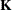matrix coefficients be displayed. These are the coefficients that apply to the LS-means. This option is useful to ensure that you assigned the coefficients correctly to the LS-means.

EXP

requests exponentiation of the least squares means estimate. When you model data with the logit link function and the estimate represents a log odds ratio, the EXP option produces an odds ratio. If you specify the CL or ALPHA= option, the (adjusted) confidence limits for the estimate are also exponentiated.

The EXP option is supported only by PROC PHREG, PROC SURVEYPHREG, the procedures that support generalized linear modeling (GENMOD, LOGISTIC, and SURVEYLOGISTIC), and by PROC PLM when it is used to perform statistical analyses on item stores that were created by these procedures.

requests that the estimate and its standard error also be reported on the scale of the mean (the inverse linked scale). The computation of the inverse linked estimate depends on the estimation mode. For example, if the analysis is based on a posterior sample when a BAYES statement is present, the inversely linked estimate is the average of the inversely linked values across the sample of posterior parameter estimates. If the analysis is not based on a sample of parameter estimates, the procedure computes the value on the mean scale by applying the inverse link to the estimate.

The interpretation of the inversely linked quantity depends on the coefficients that are specified in your LSMESTIMATE statement and the link function. For example, in a model for binary data with logit link the following LSMESTIMATE statement computes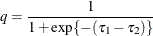where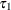and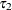are the least squares means that are associated with the first two levels of the classification effect A:

proc logistic;
class A / param=glm;
model y = A / dist=binary link=logit;
lsmestimate A 1 -1 / ilink;
run;

The quantity q is not the difference of the probabilities associated with the two levels,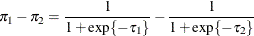The standard error of the inversely linked estimate is based on the delta method. If you also specify the CL or ALPHA= option, the procedure computes confidence intervals for the inversely linked estimate. These intervals are obtained by applying the inverse link to the confidence intervals on the linked scale.

The ILINK option is supported only by the procedures that support generalized linear modeling (GENMOD, LOGISTIC, and SURVEYLOGISTIC) and by PROC PLM when it is used to perform statistical analyses on item stores that were created by these procedures.

JOINT<(joint-test-options)>

requests that a joint F or chi-square test be produced for the rows of the estimate. For more information about the simulation-based p-value calculation, see the section Joint Hypothesis Tests with Complex Alternatives, the Chi-Bar-Square Statistic. You can specify the following joint-test-options in parentheses:

ACC=specifies the accuracy radius for determining the necessary sample size in the simulation-based approach of Silvapulle and Sen (2004) for tests with order restrictions. The value ofmust be strictly between 0 and 1; the default value is 0.005.

EPS=specifies the accuracy confidence level for determining the necessary sample size in the simulation-based approach of Silvapulle and Sen (2004) for F tests with order restrictions. The value ofmust be strictly between 0 and 1; the default value is 0.01.

LABEL='label'

assigns an identifying label to the joint test. If you do not specify a label, the first non-default label for the ESTIMATE rows is used to label the joint test.

NOEST
ONLY

performs only the joint test and suppresses other results from the ESTIMATE statement. This option is useful for emulating the CONTRAST statement that is available in other procedures.

NSAMP=n

specifies the number of samples for the simulation-based method of Silvapulle and Sen (2004). If n is not specified, it is constructed from the values of the ALPHA=, the ACC=, and the EPS=options. With the default values for,, and(0.005, 0.01, and 0.05, respectively), NSAMP=12,604 by default.

CHISQ

adds a chi-square test if the procedure produces an F test by default.

BOUNDS=value-list

specifies boundary values for the estimable linear function. The null value of the hypothesis is always zero. If you specify a positive boundary value z, the hypotheses are,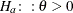with the added constraint that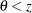. The same is true for negative boundary values. The alternative hypothesis is then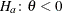subject to the constraint. If you specify a missing value, the hypothesis is assumed to be two-sided. The BOUNDS option enables you to specify sets of one- and two-sided joint hypotheses. If all values in value-list are set to missing, the procedure performs a simulation-based p-value calculation for a two-sided test.

LOWER
LOWERTAILED

requests that the p-value for the t test be based only on values that are less than the test statistic. A two-tailed test is the default. A lower-tailed confidence limit is also produced if you specify the CL or ALPHA= option.

Note that for ADJUST= SCHEFFE the one-sided adjusted confidence intervals and one-sided adjusted p-values are the same as the corresponding two-sided statistics, because this adjustment is based on only the right tail of the F distribution.

If you request an F test with the JOINT option, then a one-sided left-tailed order restriction is applied to all estimable functions, and the corresponding chi-bar-square statistic of Silvapulle and Sen (2004) is computed in addition to the two-sided, standard, F or chi-square statistic. See the JOINT option for how to control the computation of the simulation-based chi-bar-square statistic.

OBSMARGINS<=OM-data-set>
OM<=OM-data-set>

specifies a potentially different weighting scheme for the computation of LS-means coefficients. The standard LS-means have equal coefficients across classification effects; however, the OM option changes these coefficients to be proportional to those found in the OM-data-set. This adjustment is reasonable when you want your inferences to apply to a population that is not necessarily balanced but has the margins observed in OM-data-set. See the OBSMARGINS option in the LSMEANS statement for further details.

PLOTS=plot-options

produces ODS statistical graphics of the distribution of estimable functions if the procedure performs the analysis in a sampling-based mode. For example, this is the case when procedures support a BAYES statement and perform a Bayesian analysis. The estimable functions are then computed for each of the posterior parameter estimates, and the "Least Squares Means Estimates" table reports simple descriptive statistics for the evaluated functions. In this situation, the PLOTS= option enables you to visualize the distribution of the estimable function. The following plot-options are available:

ALL

produces all possible plots with their default settings.

BOXPLOT<(boxplot-options)>

produces box plots of the distribution of the estimable function across the posterior sample. A separate box plot is generated for each estimable function and all box plots appear on a single graph by default. You can affect the appearance of the box plot graph with the following options:

ORIENTATION=VERTICAL | HORIZONTAL
ORIENT=VERT | HORIZ

specifies the orientation of the boxes. The default is vertical orientation of the box plots.

NPANELPOS=number

specifies how to break the series of box plots across multiple panels. If the NPANELPOS option is not specified, or if number equals zero, then all box plots are displayed in a single graph; this is the default. If a negative number is specified, then exactly up toof box plots are displayed per panel. If number is positive, then the number of boxes per panel is balanced to achieve small variation in the number of box plots per graph.

DISTPLOT<(distplot-options)>
DIST<(distplot-options)>

generates panels of histograms with a kernel density overlaid. A separate plot in each panel contains the results for each estimable function. You can specify the following distplot-options in parentheses:

BOX | NOBOX

controls the display of a horizontal box plot below the histogram. The BOX option is enabled by default.

HIST | NOHIST

controls the display of the histogram of the estimable function’s distribution across the posterior sample. The HIST option is enabled by default.

NORMAL | NONORMAL

controls the display of a normal density estimate on the graph. The NONORMAL option is enabled by default.

KERNEL | NOKERNEL

controls the display of a kernel density estimate on the graph. The KERNEL option is enabled by default.

NROWS=number

specifies the highest number of rows in a panel. The default is 3.

NCOLS=number

specifies the highest number of columns in a panel. The default is 3.

UNPACK

unpacks the panel into separate graphics.

NONE

does not produce any plots.

SEED=number

specifies the seed for the sampling-based components of the computations for the LSMESTIMATE statement (for example, chi-bar-square statistics and simulated p-values). The value of number must be an integer. The seed is used to start the pseudo-random-number generator for the simulation. If you do not specify a seed, or if you specify a value less than or equal to zero, the seed is generated from reading the time of day from the computer clock. Note that there could be multiple LSMESTIMATE statements with SEED= specifications and there could be other statements that can supply a random number seed. Since the procedure has only one random number stream, the initial seed is shown in the SAS log.

SINGULAR=number

tunes the estimability checking as documented for the SINGULAR= option in the ESTIMATE statement.

STEPDOWN<(step-down-options)>

requests that multiplicity adjustments for the p-values of estimable functions be further adjusted in a step-down fashion. Step-down methods increase the power of multiple testing procedures by taking advantage of the fact that a p-value is never declared significant unless all smaller p-values are also declared significant. The STEPDOWN adjustment combined with ADJUST= BON corresponds to the methods of Holm (1979) and "Method 2" of Shaffer (1986); this is the default. Using step-down-adjusted p-values combined with ADJUST= SIMULATE corresponds to the method of Westfall (1997).

If the ESTIMATE statement is applied with a STEPDOWN option in a mixed model where the degrees-of-freedom method is that of Kenward and Roger (1997) or of Satterthwaite, then step-down-adjusted p-values are produced only if the ADJDFE =ROW option is in effect.

Also, the STEPDOWN option affects only p-values, not confidence limits. For ADJUST= SIMULATE, the generalized least squares hybrid approach of Westfall (1997) is used to increase Monte Carlo accuracy.

You can specify the following step-down-options in parentheses:

MAXTIME=n

specifies the time (in seconds) to be spent computing the maximal logically consistent sequential subsets of equality hypotheses for TYPE=LOGICAL. The default is MAXTIME=60. If the MAXTIME value is exceeded, the adjusted tests are not computed. When this occurs, you can try increasing the MAXTIME value. However, note that there are common multiple comparisons problems for which this computation requires a huge amount of time—for example, all pairwise comparisons between more than 10 groups. In such cases, try to use TYPE=FREE (the default) or TYPE=LOGICAL(n) for small n.

ORDER=PVALUE
ORDER=ROWS

specifies the order in which the step-down tests are performed. ORDER=PVALUE is the default, with LS-mean estimates being declared significant only if all LS-mean estimates with smaller (unadjusted) p-values are significant. If you specify ORDER=ROWS, then significances are evaluated in the order in which they are specified.

REPORT

specifies that a report on the step-down adjustment be displayed, including a listing of the sequential subsets (Westfall, 1997) and, for ADJUST= SIMULATE, the step-down simulation results.

TYPE=LOGICAL<(n)>
TYPE=FREE

specifies how step-down adjustment are made. If you specify TYPE=LOGICAL, the step-down adjustments are computed by using maximal logically consistent sequential subsets of equality hypotheses (Shaffer, 1986; Westfall, 1997). Alternatively, for TYPE=FREE, sequential subsets are computed ignoring logical constraints. The TYPE=FREE results are more conservative than those for TYPE=LOGICAL, but they can be much more efficient to produce for many estimates. For example, it is not feasible to take logical constraints between all pairwise comparisons of more than about 10 groups. For this reason, TYPE=FREE is the default.

However, you can reduce the computational complexity of taking logical constraints into account by limiting the depth of the search tree used to compute them, specifying the optional depth parameter as a number n in parentheses after TYPE=LOGICAL. As with TYPE=FREE, results for TYPE=LOGICAL(n) are conservative relative to the true TYPE=LOGICAL results. But even for TYPE=LOGICAL(0), they can be appreciably less conservative than TYPE=FREE, and they are computationally feasible for much larger numbers of estimates. If you do not specify n or if n = –1, the full search tree is used.

TESTVALUE=value-list
TESTMEAN=value-list

specifies the value under the null hypothesis for testing the estimable functions in the LSMESTIMATE statement. The rules for specifying the value-list are very similar to those for specifying the divisor list in the DIVISOR= option. If no TESTVALUE= is specified, all tests are performed as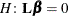. Missing values in the value-list also are translated to zeros. If you specify fewer values than rows in the LSMESTIMATE statement, the last value in value-list is carried forward.

The TESTVALUE= option affects only p-values from individual, joint, and multiplicity-adjusted tests. It does not affect confidence intervals.

The TESTVALUE option is not available for the multinomial distribution, and the values are ignored when you perform a sampling-based (Bayesian) analysis.

UPPER
UPPERTAILED

requests that the p-value for the t test be based only on values that are greater than the test statistic. A two-tailed test is the default. An upper-tailed confidence limit is also produced if you specify the CL or ALPHA= option.

Note that for ADJUST= SCHEFFE the one-sided adjusted confidence intervals and one-sided adjusted p-values are the same as the corresponding two-sided statistics, because this adjustment is based on only the right tail of the F distribution.

If you request a joint test with the JOINT option, then a one-sided right-tailed order restriction is applied to all estimable functions, and the corresponding chi-bar-square statistic of Silvapulle and Sen (2004) is computed in addition to the two-sided, standard, F or chi-square statistic. See the JOINT option for how to control the computation of the simulation-based chi-bar-square statistic.